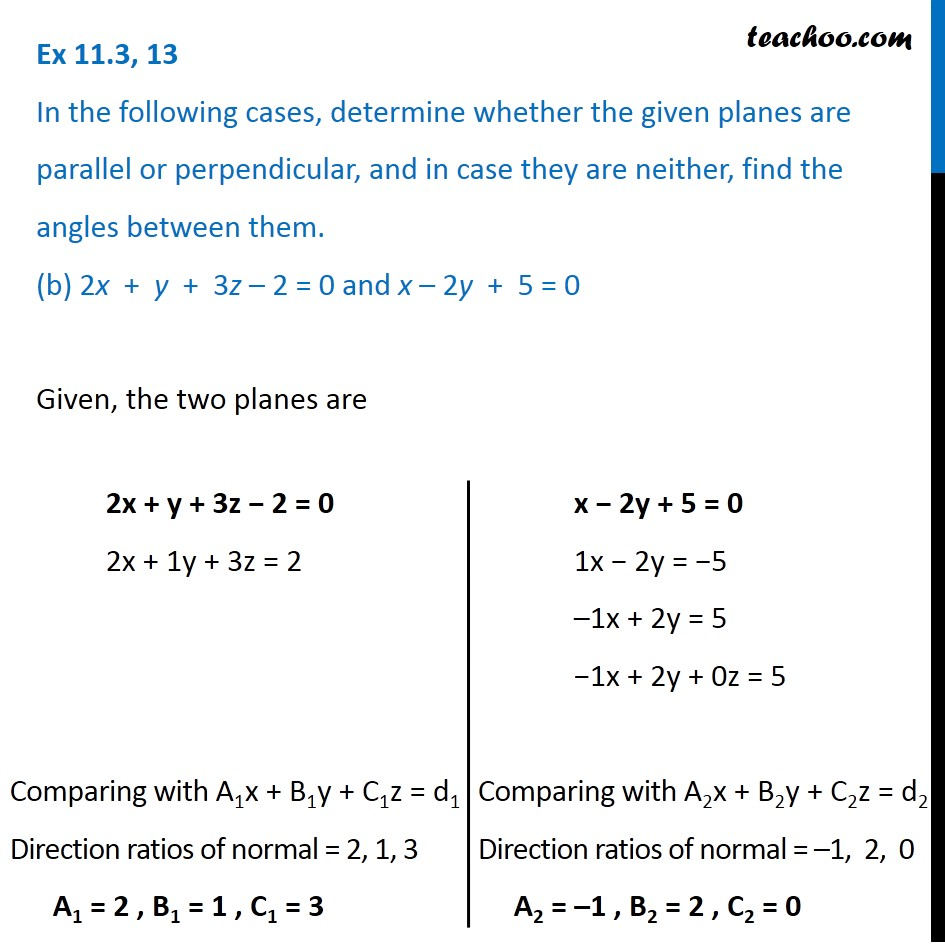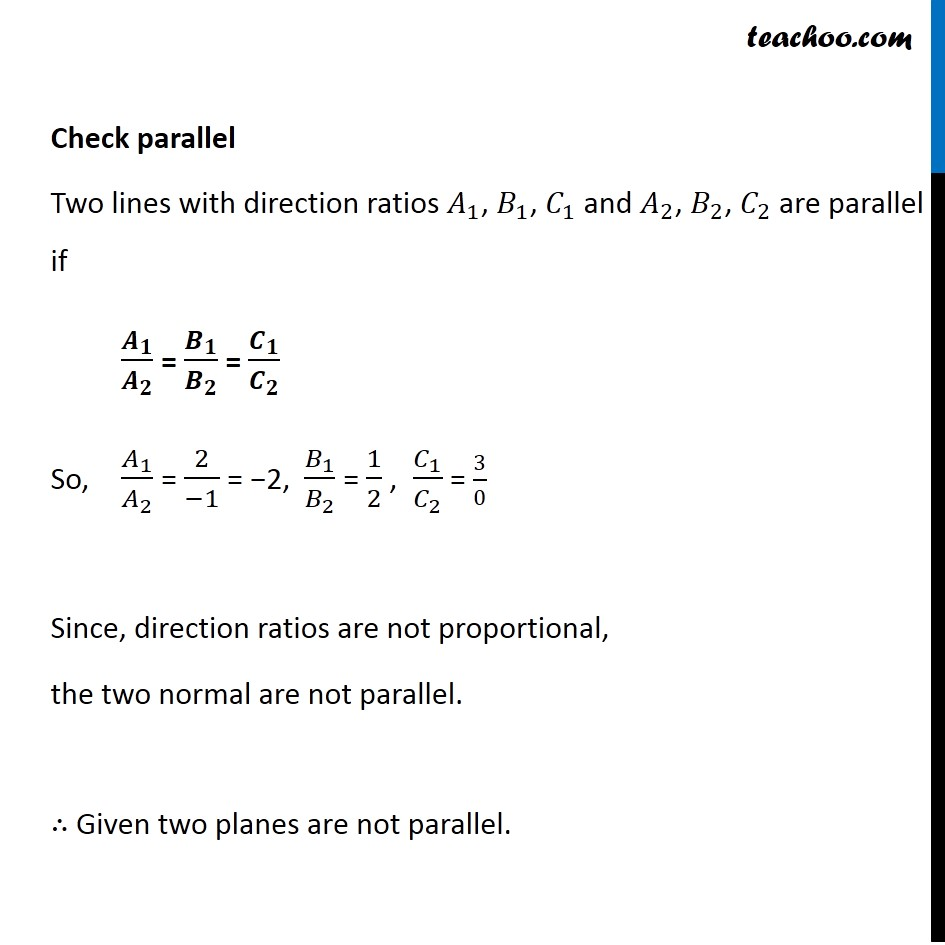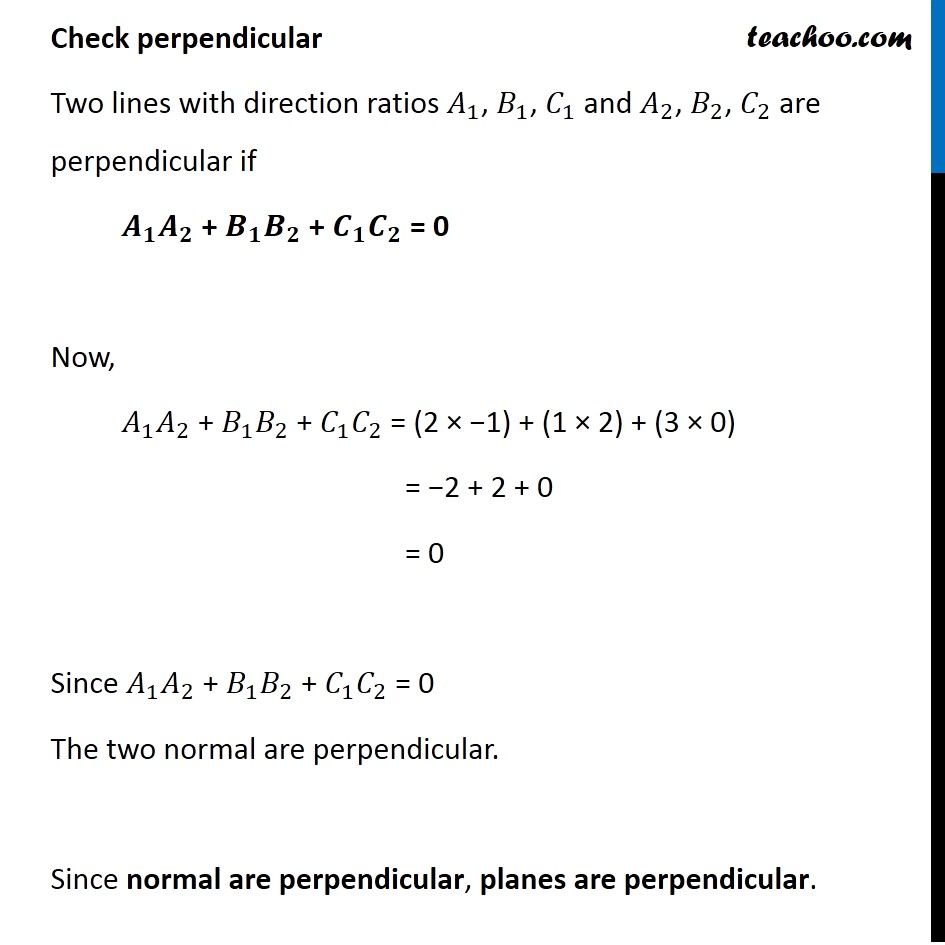Plane

Chapter 11 Class 12 Three Dimensional Geometry
Serial order wiseLearn in your speed, with individual attention - Teachoo Maths 1-on-1 Class

### Transcript

Question 13 In the following cases, determine whether the given planes are parallel or perpendicular, and in case they are neither, find the angles between them. (b) 2x + y + 3z – 2 = 0 and x – 2y + 5 = 0 Check parallel Two lines with direction ratios 𝐴_1, 𝐵_1, 𝐶_1 and 𝐴_2, 𝐵_2, 𝐶_2 are parallel if 𝑨_𝟏/𝑨_𝟐 = 𝑩_𝟏/𝑩_𝟐 = 𝑪_𝟏/𝑪_𝟐 So, 𝐴_1/𝐴_2 = 2/(−1) = −2, 𝐵_1/𝐵_2 = 1/2 , 𝐶_1/𝐶_2 = 3/0 Since, direction ratios are not proportional, the two normal are not parallel. ∴ Given two planes are not parallel. Check perpendicular Two lines with direction ratios 𝐴_1, 𝐵_1, 𝐶_1 and 𝐴_2, 𝐵_2, 𝐶_2 are perpendicular if 𝑨_𝟏 𝑨_𝟐 + 𝑩_𝟏 𝑩_𝟐 + 𝑪_𝟏 𝑪_𝟐 = 0 Now, 𝐴_1 𝐴_2 + 𝐵_1 𝐵_2 + 𝐶_1 𝐶_2 = (2 × −1) + (1 × 2) + (3 × 0) = −2 + 2 + 0 = 0 Since 𝐴_1 𝐴_2 + 𝐵_1 𝐵_2 + 𝐶_1 𝐶_2 = 0 The two normal are perpendicular. Since normal are perpendicular, planes are perpendicular.